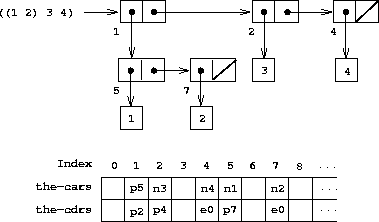# Box-and-pointer and memory-vector representations

Draw the box-and-pointer representation and the memory-vector representation (as in figure 5.14) of the list structure produced by

``````(define x (cons 1 2))

(define y (list x x))
``````

with the `free` pointer initially `p1` . What is the final value of `free` ? What pointers represent the values of `x` and `y` ?Figure 5.14: Box-and-pointer and memory-vector representations of the list ((1 2) 3 4).